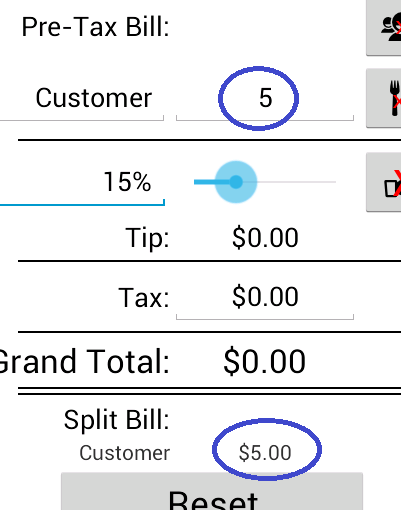glaukon
Chapter 3: Java - Part 13
Once we have that, we set tvSplitBill using String.format("%,.2f", total). This is a complicated method. The String.format method is used to both turn things into strings, as well as format them. It takes 2 arguments. The second is the thing we want formatted, which, in this case, is the double called total. The first argument is a string that represents the rules for formatting the second argument. I won't go into the details of all the rules. You can research it on your own. The glossary link would be a good place to start. But I will go over what %,.2f in particular means, since it's a set of formatting rules we'll be using a lot.
There are four parts here. The first part is %. For the purposes of this app, just know that formatting rules have to start with %, and everything after it represents the actual rules. The next part is ,. This is a flag. Flags are used to tell the computer the number should have certain quirks. The , flag tells the computer to insert commas into the number according to the norms of whatever locale the user's device is set to. For instance, in the United States, the computer will put commas to denote thousands. The third part is .2. This part defines precision. By putting .2, we're telling the computer to format the number to 2 decimal places. So if the number is 5, we'll get 5.00. If the number is 5.339, we'll get 5.34 (yes, it rounds). If you want 3 decimal places, just change the precision to .3. The same goes for any other number of decimal places. Lastly, the fourth part is f. This part denotes the conversion, or type. The f type tells the computer that the number to be formatted is a floating point number, which just means it can have fractional amounts, and doesn't have to be a whole number. If you just wanted to format an integer, you can use the d type, which stands for decimal, and, for some reason, refers to non-floating point numbers.
Let's re-cap the String.format method. The first argument it takes defines the formatting rules we want. %,.2f means we want to use commas in our number, we want it to have 2 decimal places, and the number is a floating point number. The second argument is the thing we want formatted. Based on what I've told you, you can experiment with String.format some more. Note that generally, the only thing you actually have to specify in the first argument to use this method is the type. Everything else is optional. But if you want to use f as the type, you'll also at least need to specify precision. The order you specify the rules does matter, and there are other kinds of rules that we aren't using here. Again, if you're curious, you should do some more research on your own.
Okay, that took longer than I wanted it to, but let's move on. So we format total, which is a double, to a string with commas and 2 decimal places. We then add a dollar sign in front of it with "\$" +. Yes, we can add strings together, just like we can add integers and doubles. I can't remember if I mentioned that before, since its been over a month since I started writing this tutorial, but ya, just fyi.
Wow, okay then, we're done with this for now. The updateTotal method sets the total to 0, then sets it equal to the sum of all the orders of the Diner. Then it sets the Diner's tvSplitBill TextView to the total. Basically. Okay, let's test. Run this in an AVD, and change the first Diner's first order to 5. Then click out of the EditText by selecting a different EditText. You should see this:Glossary
Conversion: The conversion, or type, part of the String.format method is mandatory. The method doesn't work if you don't set this. It refers to what kind of object is being formatted (floating point number, whole number, text, etc.).
Flag: Flags are used in the String.format method to define a set of rules to format something into a string.
Precision: The precision part of the String.format method essentially sets how many characters the resulting string will have (i.e. how long, or, in number terms, how precise it will be).
String.format(String format, Object args): A method you can use on an object (second argument) to turn it into a string, and format it based on a set of rules summarized by a short string of characters (first argument).